# Types of Chemical Reactions and Solution Stoichiometry Chapter

• Slides: 35Types of Chemical Reactions and Solution Stoichiometry Chapter 4 E-mail: benzene 4 [email protected] com Web-site: http: //clas. sa. ucsb. edu/staff/terri/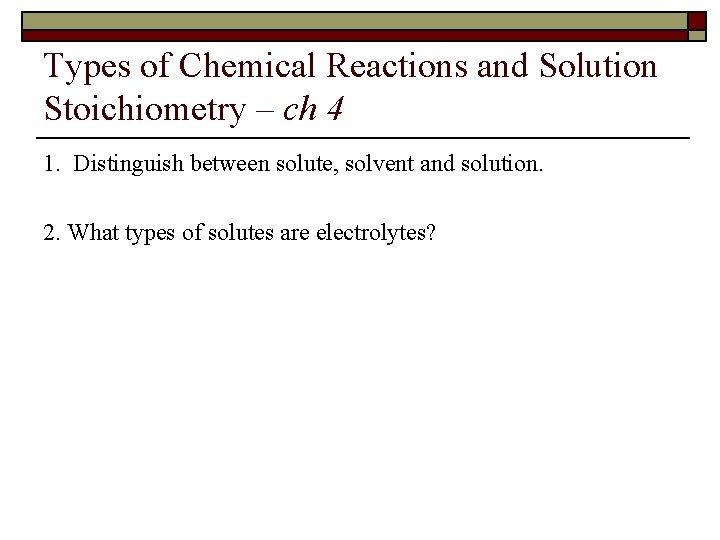Types of Chemical Reactions and Solution Stoichiometry – ch 4 1. Distinguish between solute, solvent and solution. 2. What types of solutes are electrolytes?Types of Chemical Reactions and Solution Stoichiometry – ch 4 Dissolved ionic (Na. Cl) Electrolyte Solution Dissolved molecules (Sugar) Non-electrolyte Solution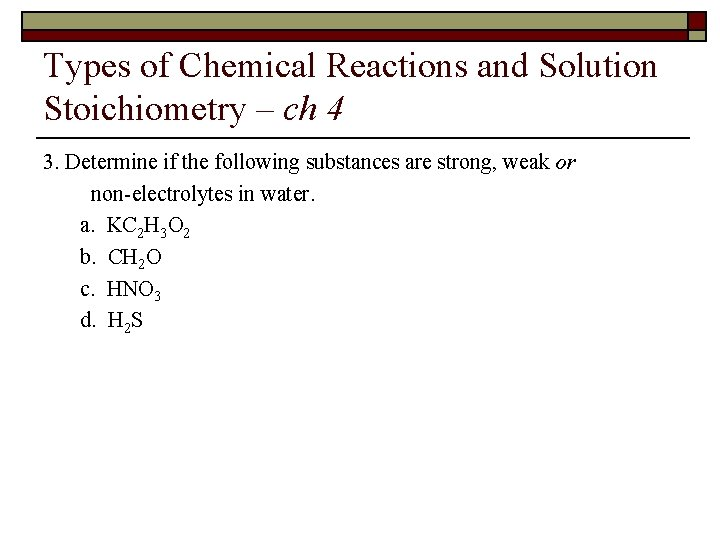Types of Chemical Reactions and Solution Stoichiometry – ch 4 3. Determine if the following substances are strong, weak or non-electrolytes in water. a. KC 2 H 3 O 2 b. CH 2 O c. HNO 3 d. H 2 S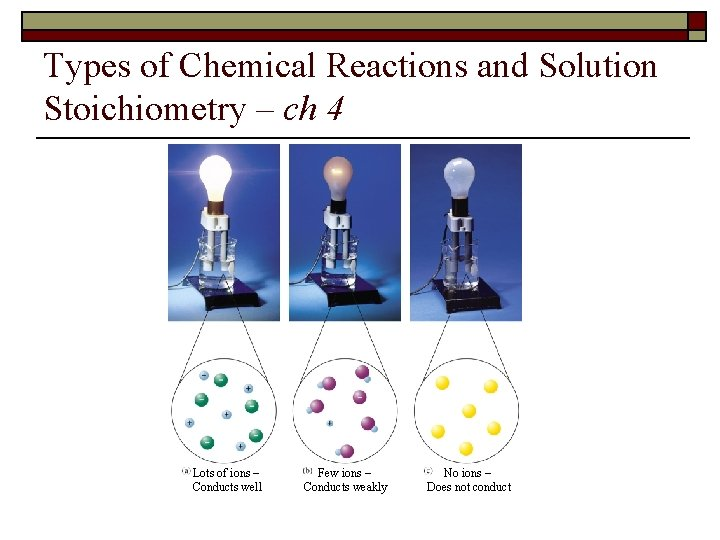Types of Chemical Reactions and Solution Stoichiometry – ch 4 Lots of ions – Conducts well Few ions – Conducts weakly No ions – Does not conduct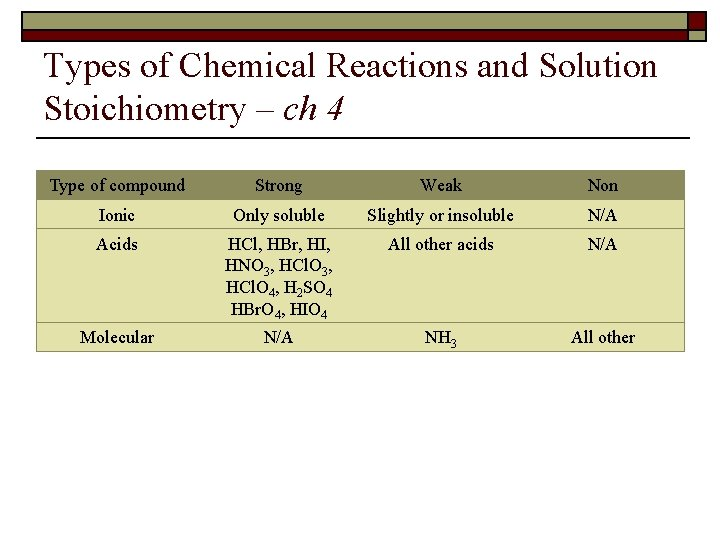Types of Chemical Reactions and Solution Stoichiometry – ch 4 Type of compound Strong Weak Non Ionic Only soluble Slightly or insoluble N/A Acids HCl, HBr, HI, HNO 3, HCl. O 3, HCl. O 4, H 2 SO 4 HBr. O 4, HIO 4 All other acids N/A Molecular N/A NH 3 All otherTypes of Chemical Reactions and Solution Stoichiometry – ch 4 4. A solution is prepared by dissolving 50 g of barium fluoride in enough water to make 750 m. L of solution. a. What is the molarity of the barium fluoride? b. What is the concentration for each of the ions in solution? c. What is the concentration of the fluoride ion if 350 m. L of water is added?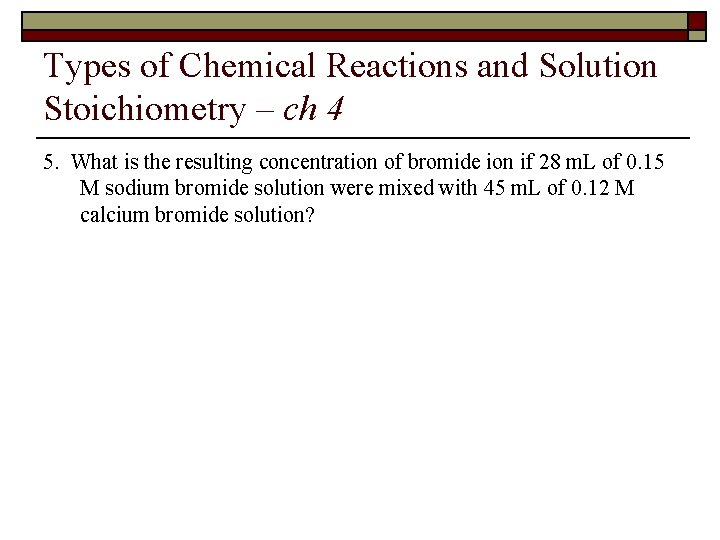Types of Chemical Reactions and Solution Stoichiometry – ch 4 5. What is the resulting concentration of bromide ion if 28 m. L of 0. 15 M sodium bromide solution were mixed with 45 m. L of 0. 12 M calcium bromide solution?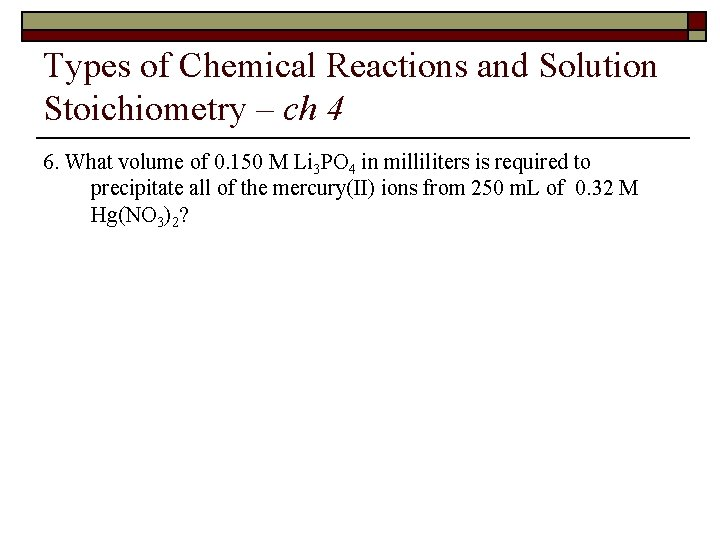Types of Chemical Reactions and Solution Stoichiometry – ch 4 6. What volume of 0. 150 M Li 3 PO 4 in milliliters is required to precipitate all of the mercury(II) ions from 250 m. L of 0. 32 M Hg(NO 3)2?Types of Chemical Reactions and Solution Stoichiometry – ch 4 7. A precipitate will form when 10 m. L of 0. 25 M calcium chloride is mixed with 8 m. L of 0. 4 M silver nitrate. a. Write the molecular and net ionic equation for this reaction b. How many grams of precipitate form? c. What is the concentration of the ions in the solution after the reaction has gone to completion?Types of Chemical Reactions and Solution Stoichiometry – ch 4 8. What type of solution results (acidic, basic or neutral) when 110 m. L of 0. 40 M HC 2 H 3 O 2 is mixed with 42 m. L of 0. 90 M Ba(OH)2? Write the molecular and net ionic equations.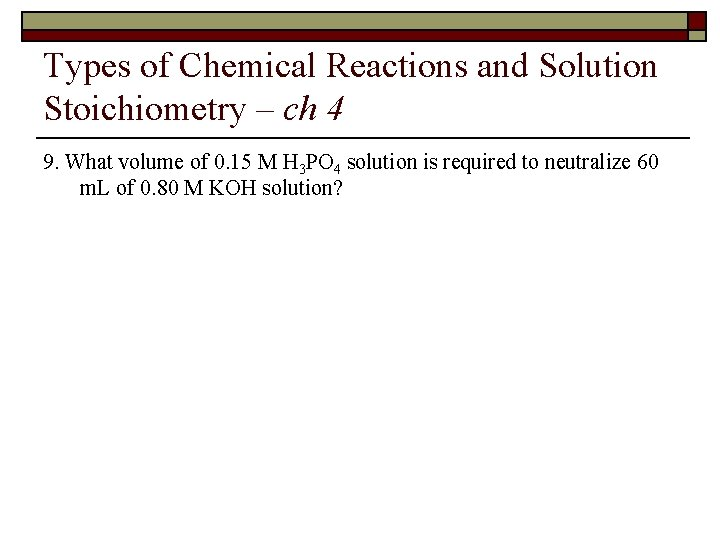Types of Chemical Reactions and Solution Stoichiometry – ch 4 9. What volume of 0. 15 M H 3 PO 4 solution is required to neutralize 60 m. L of 0. 80 M KOH solution?Types of Chemical Reactions and Solution Stoichiometry – ch 4 10. Assign oxidation states for the following: a. S in SO 3 b. P in PF 5 c. Cl in Cl 2 d. C in C 3 H 8 e. Mn in Mn. O 4– f. Cr in K 2 Cr 2 O 7 g. N in NH 4 NO 3Types of Chemical Reactions and Solution Stoichiometry – ch 4 Rules and Hierarchy for Assigning Oxidation Numbers/States 1. What type of substance are you analyzing? a. Element ⇒ The oxidation state for atoms in their elemental form are 0 b. Molecular Compound ⇒ the sum of the oxidation numbers in a compound equals 0 c. Ionic compound ⇒ analyze the cation and anion individually - Mono-atomic ions ⇒ The oxidation state for mono-atomic ions is the charge on the ion - Polyatomic ions ⇒ The sum of the oxidation numbers in a polyatomic ion equals the net charge on the ion 2. The hierarchy tells which element gets assigned first: Hierarchy of Substances Hydrogen in a molecular compound or polyatomic ion Fluorine in a molecular compound Oxygen in a molecular compound or polyatomic ion Chlorine, bromine or iodine in a molecular Oxidation State +1 -1 -2 -1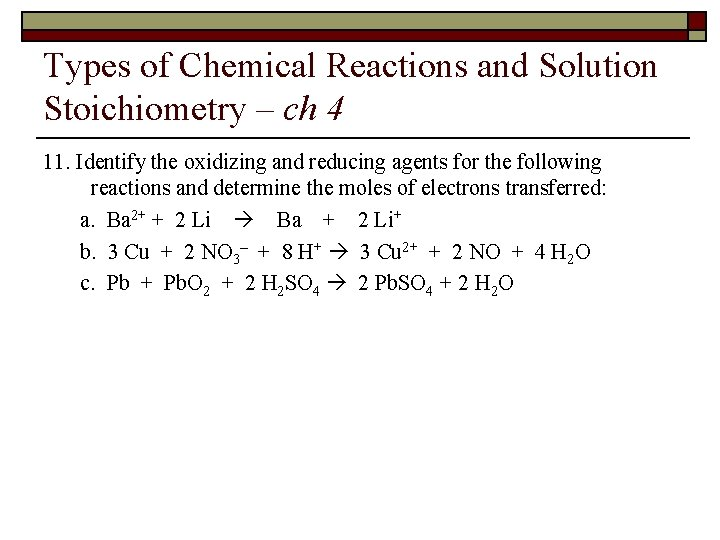Types of Chemical Reactions and Solution Stoichiometry – ch 4 11. Identify the oxidizing and reducing agents for the following reactions and determine the moles of electrons transferred: a. Ba 2+ + 2 Li Ba + 2 Li+ b. 3 Cu + 2 NO 3– + 8 H+ 3 Cu 2+ + 2 NO + 4 H 2 O c. Pb + Pb. O 2 + 2 H 2 SO 4 2 Pb. SO 4 + 2 H 2 OTypes of Chemical Reactions and Solution Stoichiometry – ch 4 1. 2. 3. 4. 5. 6. Oxidation-Reduction (Redox) Reactions A reaction in which there is a transfer of electrons Oxidation is when an element loses electrons and is noted by an increase in the oxidation number Reduction is when an element gains electrons and is noted by a decrease in the oxidation number The number of electrons gained must equal the number of electrons lost An oxidizing agent is the substance getting reduced A reducing agent is the substance getting oxidized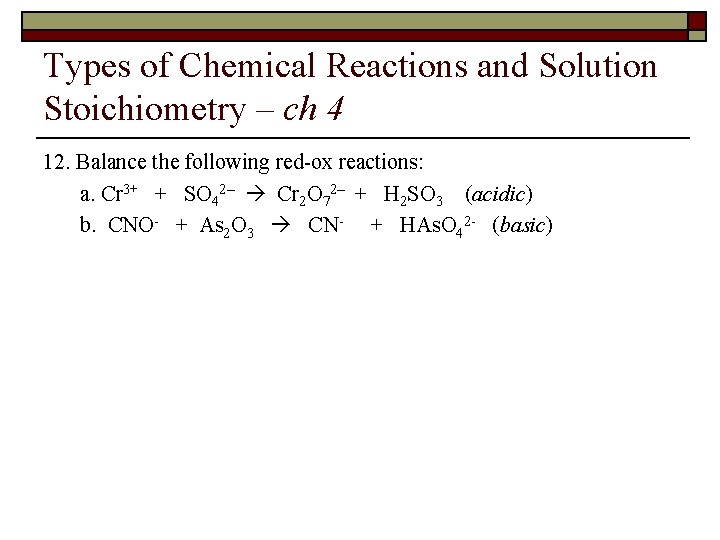Types of Chemical Reactions and Solution Stoichiometry – ch 4 12. Balance the following red-ox reactions: a. Cr 3+ + SO 42– Cr 2 O 72– + H 2 SO 3 (acidic) b. CNO- + As 2 O 3 CN- + HAs. O 42 - (basic)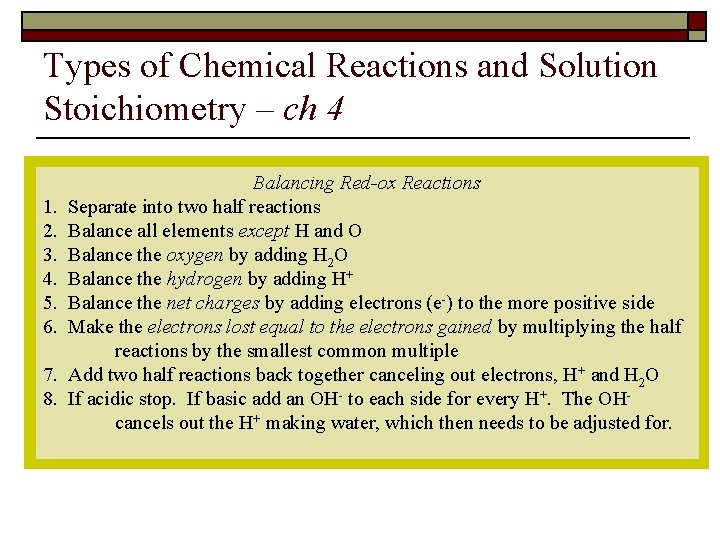Types of Chemical Reactions and Solution Stoichiometry – ch 4 Balancing Red-ox Reactions 1. Separate into two half reactions 2. Balance all elements except H and O 3. Balance the oxygen by adding H 2 O 4. Balance the hydrogen by adding H+ 5. Balance the net charges by adding electrons (e-) to the more positive side 6. Make the electrons lost equal to the electrons gained by multiplying the half reactions by the smallest common multiple 7. Add two half reactions back together canceling out electrons, H+ and H 2 O 8. If acidic stop. If basic add an OH- to each side for every H+. The OH- cancels out the H+ making water, which then needs to be adjusted for.Types of Chemical Reactions and Solution Stoichiometry – ch 4 13. The reaction below can be used as a laboratory method of preparing small quantities of Cl 2(g). If a 62. 6 g sample that is 89. 5% K 2 Cr 2 O 7 by mass is allowed to react with 225 m. L of HCl(aq) with a density of 1. 15 g/m. L and 30. 1% HCl by mass, how many grams of Cl 2(g) are produced? Cr 2 O 72 - (aq) + Cl-(aq) Cr 3+(aq) + Cl 2(g) (unbalanced)Types of Chemical Reactions and Solution Stoichiometry – ch 4 You have completed ch. 4Answer Key – Ch. 4 1. Distinguish between solute, solvent and solution. solute – a substance dissolved (typically into a liquid) to form a solution solvent – the dissolving medium in a solution – homogenous mixture of solute and solvent or 2 solvents 2. What is an electrolyte? electrolyte – a substance that produces ions upon dissolving in water thus causing a solution that can conduct electricity – all ionic compounds and acids are electrolytes – only some molecular are electrolytesAnswer Key – Ch. 4 3. Determine if the following substances are strong, weak or non electrolytes in water. a. KC 2 H 3 O 2 ⇒ soluble ionic ⇒ strong electrolyte b. CH 2 O ⇒ molecular ⇒ non electrolyte c. HNO 3 ⇒ strong acid ⇒ strong electrolyte d. H 2 S ⇒ weak acid ⇒ weak electrolyte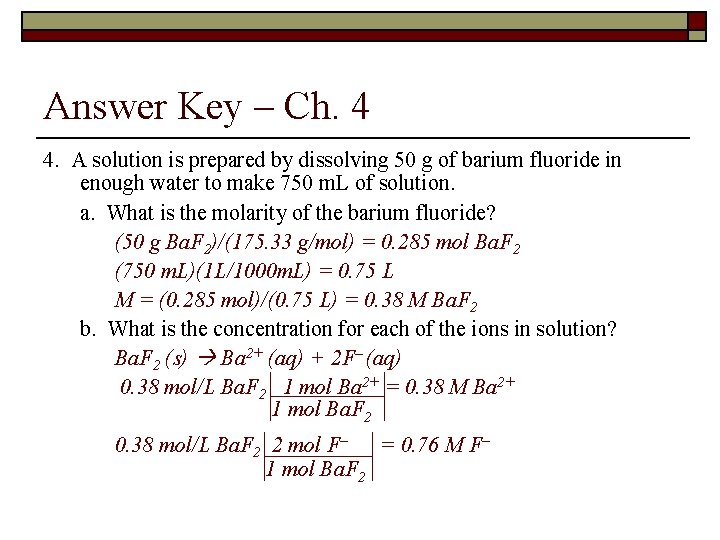Answer Key – Ch. 4 4. A solution is prepared by dissolving 50 g of barium fluoride in enough water to make 750 m. L of solution. a. What is the molarity of the barium fluoride? (50 g Ba. F 2)/(175. 33 g/mol) = 0. 285 mol Ba. F 2 (750 m. L)(1 L/1000 m. L) = 0. 75 L M = (0. 285 mol)/(0. 75 L) = 0. 38 M Ba. F 2 b. What is the concentration for each of the ions in solution? Ba. F 2 (s) Ba 2+ (aq) + 2 F– (aq) 0. 38 mol/L Ba. F 2 1 mol Ba 2+ = 0. 38 M Ba 2+ 1 mol Ba. F 2 0. 38 mol/L Ba. F 2 2 mol F– = 0. 76 M F– 1 mol Ba. F 2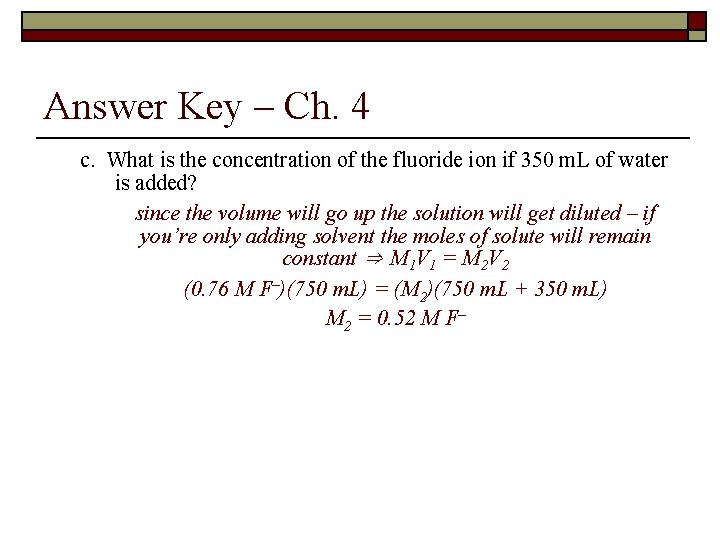Answer Key – Ch. 4 c. What is the concentration of the fluoride ion if 350 m. L of water is added? since the volume will go up the solution will get diluted – if you’re only adding solvent the moles of solute will remain constant ⇒ M 1 V 1 = M 2 V 2 (0. 76 M F–)(750 m. L) = (M 2)(750 m. L + 350 m. L) M 2 = 0. 52 M F–Answer Key – Ch. 4 5. What is the resulting concentration of bromide ion if 28 m. L of 0. 15 M sodium bromide solution were mixed with 45 m. L of 0. 12 M calcium bromide solution? Na. Br (s) Na+ (aq) + Br– (aq) 0. 15 M Na. Br ⇒ 0. 15 M Br– (0. 15 mol/L)(28 m. L) = 4. 2 mmol Br– (before mixing) Ca. Br 2 (s) Ca 2+ (aq) + 2 Br– (aq) 0. 12 M Ca. Br 2 ⇒ 0. 24 M Br– (0. 24 mol/L)(45 m. L) = 10. 8 mmol Br– (before mixing) After mixing ⇒ 4. 2 mmol Br– + 10. 8 mmol = 15 mmol Br– Final concentration ⇒ (15 mmol Br– )/(28 m. L + 45 m. L) = 0. 21 M Br–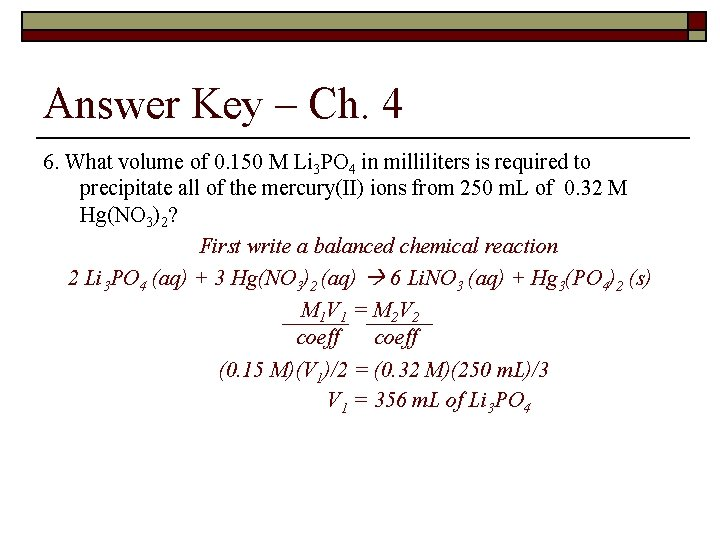Answer Key – Ch. 4 6. What volume of 0. 150 M Li 3 PO 4 in milliliters is required to precipitate all of the mercury(II) ions from 250 m. L of 0. 32 M Hg(NO 3)2? First write a balanced chemical reaction 2 Li 3 PO 4 (aq) + 3 Hg(NO 3)2 (aq) 6 Li. NO 3 (aq) + Hg 3(PO 4)2 (s) M 1 V 1 = M 2 V 2 coeff (0. 15 M)(V 1)/2 = (0. 32 M)(250 m. L)/3 V 1 = 356 m. L of Li 3 PO 4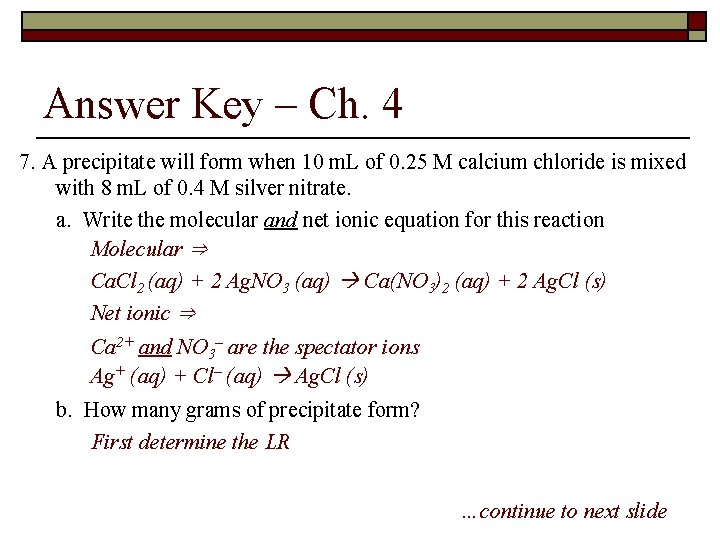Answer Key – Ch. 4 7. A precipitate will form when 10 m. L of 0. 25 M calcium chloride is mixed with 8 m. L of 0. 4 M silver nitrate. a. Write the molecular and net ionic equation for this reaction Molecular ⇒ Ca. Cl 2 (aq) + 2 Ag. NO 3 (aq) Ca(NO 3)2 (aq) + 2 Ag. Cl (s) Net ionic ⇒ Ca 2+ and NO 3– are the spectator ions Ag+ (aq) + Cl– (aq) Ag. Cl (s) b. How many grams of precipitate form? First determine the LR …continue to next slide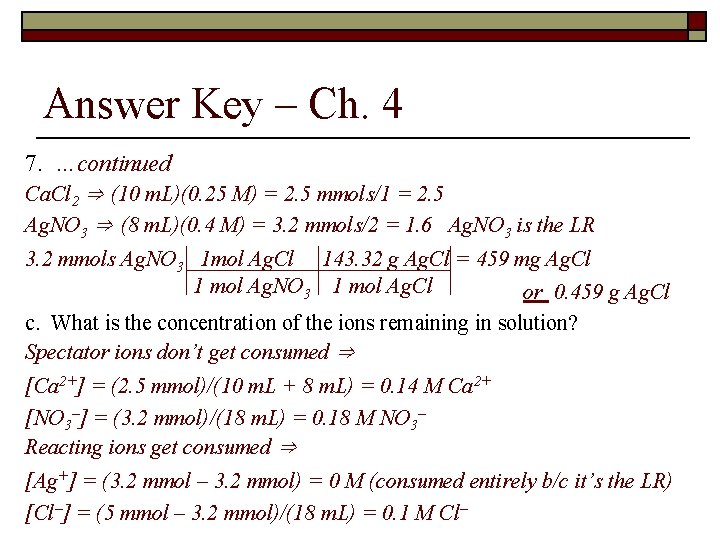Answer Key – Ch. 4 7. …continued Ca. Cl 2 ⇒ (10 m. L)(0. 25 M) = 2. 5 mmols/1 = 2. 5 Ag. NO 3 ⇒ (8 m. L)(0. 4 M) = 3. 2 mmols/2 = 1. 6 Ag. NO 3 is the LR 3. 2 mmols Ag. NO 3 1 mol Ag. Cl 143. 32 g Ag. Cl = 459 mg Ag. Cl 1 mol Ag. NO 3 1 mol Ag. Cl or 0. 459 g Ag. Cl c. What is the concentration of the ions remaining in solution? Spectator ions don’t get consumed ⇒ [Ca 2+] = (2. 5 mmol)/(10 m. L + 8 m. L) = 0. 14 M Ca 2+ [NO 3–] = (3. 2 mmol)/(18 m. L) = 0. 18 M NO 3– Reacting ions get consumed ⇒ [Ag+] = (3. 2 mmol – 3. 2 mmol) = 0 M (consumed entirely b/c it’s the LR) [Cl–] = (5 mmol – 3. 2 mmol)/(18 m. L) = 0. 1 M Cl–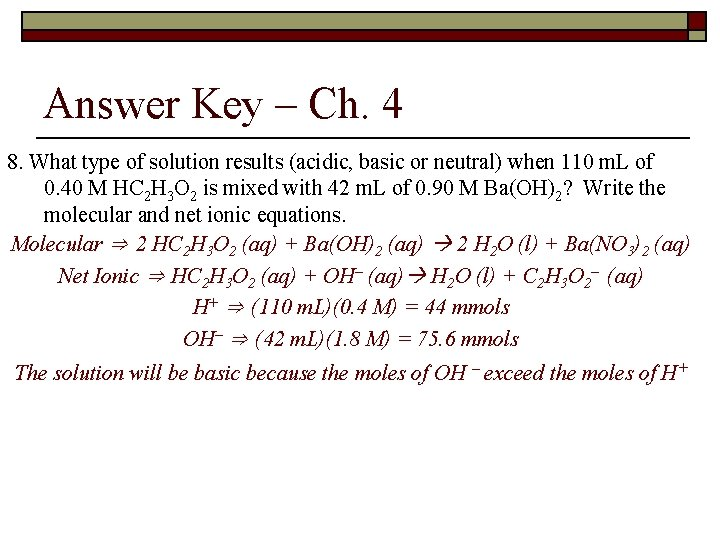Answer Key – Ch. 4 8. What type of solution results (acidic, basic or neutral) when 110 m. L of 0. 40 M HC 2 H 3 O 2 is mixed with 42 m. L of 0. 90 M Ba(OH)2? Write the molecular and net ionic equations. Molecular ⇒ 2 HC 2 H 3 O 2 (aq) + Ba(OH)2 (aq) 2 H 2 O (l) + Ba(NO 3)2 (aq) Net Ionic ⇒ HC 2 H 3 O 2 (aq) + OH– (aq) H 2 O (l) + C 2 H 3 O 2– (aq) H+ ⇒ (110 m. L)(0. 4 M) = 44 mmols OH– ⇒ (42 m. L)(1. 8 M) = 75. 6 mmols The solution will be basic because the moles of OH – exceed the moles of H+Answer Key – Ch. 4 9. What volume of 0. 15 M H 3 PO 4 is required to neutralize 60 m. L of 0. 80 M KOH? H 3 PO 4 + 3 KOH 3 H 2 O + K 3 PO 4 M 1 V 1 = M 2 V 2 Coeff (0. 15 M)(V 1)/1 = (0. 8 M)(60 m. L)/3 V 1 = 107 m. L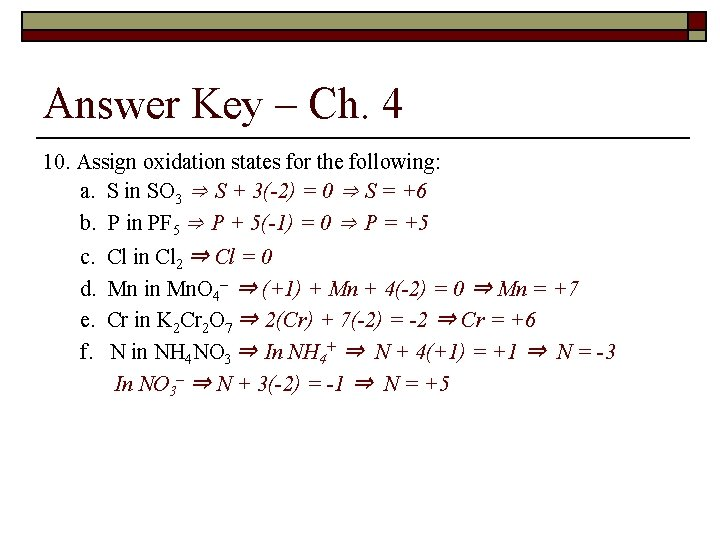Answer Key – Ch. 4 10. Assign oxidation states for the following: a. S in SO 3 ⇒ S + 3(-2) = 0 ⇒ S = +6 b. P in PF 5 ⇒ P + 5(-1) = 0 ⇒ P = +5 c. Cl in Cl 2 ⇒ Cl = 0 d. Mn in Mn. O 4– ⇒ (+1) + Mn + 4(-2) = 0 ⇒ Mn = +7 e. Cr in K 2 Cr 2 O 7 ⇒ 2(Cr) + 7(-2) = -2 ⇒ Cr = +6 f. N in NH 4 NO 3 ⇒ In NH 4+ ⇒ N + 4(+1) = +1 ⇒ N = -3 In NO 3– ⇒ N + 3(-2) = -1 ⇒ N = +5Answer Key – Ch. 4 11. Identify the oxidizing and reducing agents for the following reactions and determine the moles of electrons transferred: a. Ba 2+ + 2 Li Ba + 2 Li+ Ba 2+ is getting reduced ⇒ oxidizing agent Li is getting oxidized ⇒ reducing agent 2 moles of electrons b. 3 Cu + 2 NO 3– + 8 H+ 3 Cu 2+ + 2 NO + 4 H 2 O Cu is getting oxidized reducing agent N in NO 3– is getting reduced oxidizing agent 6 moles of electrons c. Pb + Pb. O 2 + 2 H 2 SO 4 2 Pb. SO 4 + 2 H 2 O Pb is getting oxidized reducing agent Pb in Pb. O 2 is getting reduced oxidizing agent 2 moles of electrons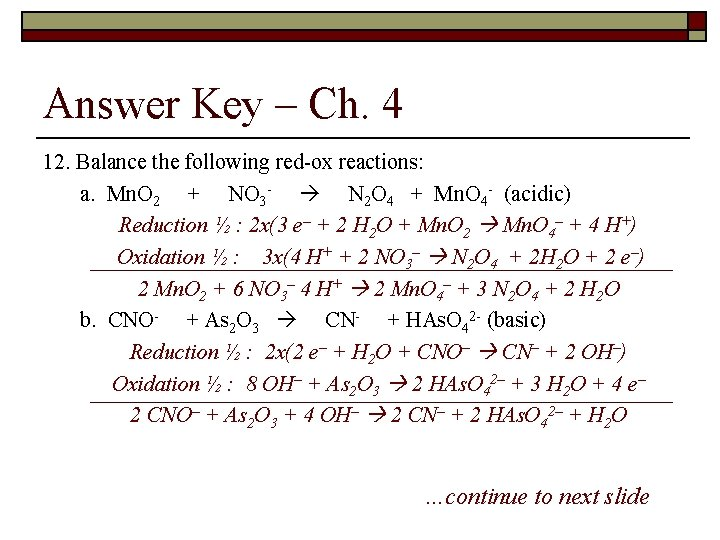Answer Key – Ch. 4 12. Balance the following red-ox reactions: a. Mn. O 2 + NO 3 - N 2 O 4 + Mn. O 4 - (acidic) Reduction ½ : 2 x(3 e– + 2 H 2 O + Mn. O 2 Mn. O 4– + 4 H+) Oxidation ½ : 3 x(4 H+ + 2 NO 3– N 2 O 4 + 2 H 2 O + 2 e–) 2 Mn. O 2 + 6 NO 3– 4 H+ 2 Mn. O 4– + 3 N 2 O 4 + 2 H 2 O b. CNO- + As 2 O 3 CN- + HAs. O 42 - (basic) Reduction ½ : 2 x(2 e– + H 2 O + CNO– CN– + 2 OH–) Oxidation ½ : 8 OH– + As 2 O 3 2 HAs. O 42– + 3 H 2 O + 4 e– 2 CNO– + As 2 O 3 + 4 OH– 2 CN– + 2 HAs. O 42– + H 2 O …continue to next slide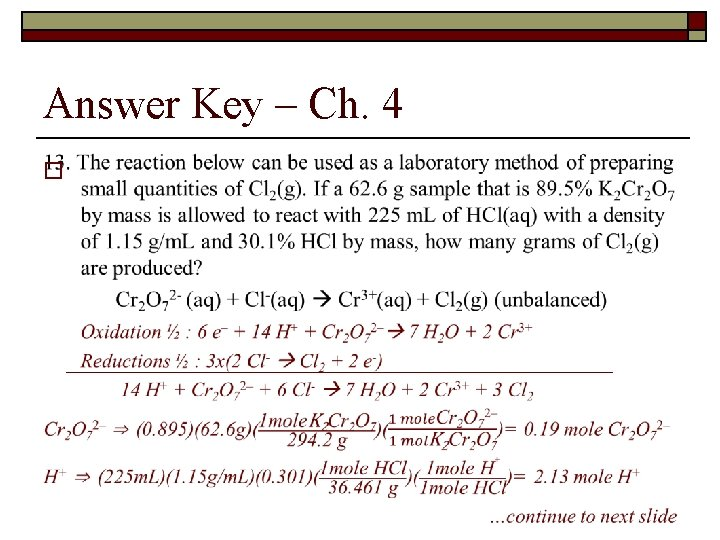Answer Key – Ch. 4 o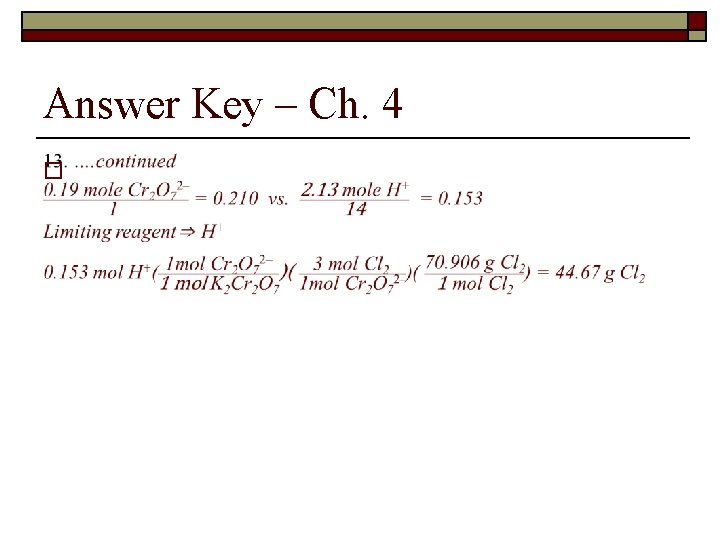Answer Key – Ch. 4 o